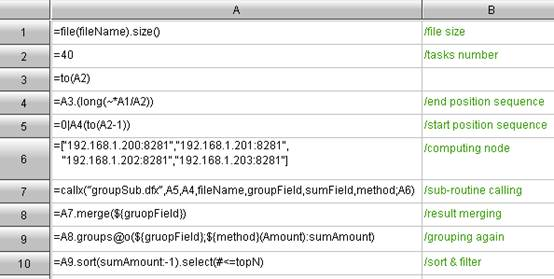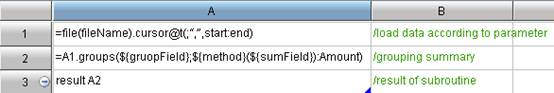This week's book giveaway is in the Java in General forum.We're giving away four copies of Event Streams in Action and have Alexander Dean & Valentin Crettaz on-line!See this thread for details.Win a copy of Event Streams in Action this week in the Java in General forum!
programming forums Java Mobile Certification Databases Caching Books Engineering Micro Controllers OS Languages Paradigms IDEs Build Tools Frameworks Application Servers Open Source This Site Careers Other all forums
this forum made possible by our volunteer staff, including ...
Marshals:
• Campbell Ritchie
• Devaka Cooray
• Liutauras Vilda
• Jeanne Boyarsky
• Bear Bibeault
Sheriffs:
• Paul Clapham
• Knute Snortum
• Rob Spoor
Saloon Keepers:
• Tim Moores
• Ron McLeod
• Piet Souris
• Stephan van Hulst
• Carey Brown
Bartenders:
• Tim Holloway
• Frits Walraven
• Ganesh Patekar

# Agile program language to deal with complex procedures

Greenhorn
Posts: 11••••Hadoop is an outstanding parallel computing system whose default parallel computing mode is MapReduce. However, such parallel computing is not specially designed for parallel data computing. Plus, it is not an agile parallel computing program language, the coding efficiency for data computing is relatively low, and this parallel computing is even more difficult to compose the universal algorithm.

Regarding the agile program language and parallel computing, esProc and MapReduce are very similar in function.

Here is an example illustrating how to develop parallel computing in Hadoop with an agile program language. Take the common Group algorithm in MapReduce for example: According to the order data on HDFS, sum up the sales amount of sales person, and seek the top N salesman. In the example code of agile program language, the big data file fileName, fields-to-group groupField, fileds-to-summarizing sumField, syntax-for-summarizing method, and the top-N-list topN are all parameters. In esProc, the corresponding agile program language codes are shown below:

Agile program language code for summary machine:Agile program language code for node machine:How to perform the parallel data computing over big data? The most intuitive idea occurs to you would be: Decompose a task into several parallel segments to conduct parallel computing; distribute them to the unit machine to summarize initially; and then further summarize the summary machine for the second time.

From the above codes, we can see that esProc has parallel data computing into two categories: The respective codes for summary machine and node machine. The summary machine is responsible for task scheduling, distributing the task to every parallel computing node in the form of parameter to conduct parallel computing, and ultimately consolidating and summarizing the parallel computing results from parallel computing node machines. The node machines are used to get a segment of the whole data piece as specified by parameters, and then group and summarize the data of this segment.

Then, let’s discuss the above-mentioned parallel data computingcodes in details.
Variable definition in parallel computing

As can be seen from the above parallel computing codes, esProc is the codes written in the cells. Each cell is represented with a unique combination of row ID and column ID. The variable is the cell name requiring no definition, for example, in the summary machine code:
 A2: =40
 A6: = ["192. 168. 1. 200: 8281","192. 168. 1. 201: 8281","192. 168. 1. 202: 8281","192. 168. 1. 203: 8281"]
A2 and A6 are just two variables representing the number of parallel computing tasks and the list of node machines respectively. The other agile program language codes can reference the variables with the cell name directly. For example, the A3, A4, and A5 all reference A2, and A7 references A6.

Since the variable is itself the cell name, the reference between cells is intuitive and convenient. Obviously, this parallel computing method allows for decomposing a great goal into several simple parallel computing steps, and achieving the ultimate goal by invoking progressively between steps. In the above codes: A8 makes references to A7, A9 references the A8, and A9 references A10. Each step is aimed to solve a small problem in parallel computing. Step by step, the parallel computing goal of this example is ultimately solved.

External parameter in parallel computing

In esProc, a parameter can be used as the normal parameter or macro. For example, in the agile program language code of summary machine, the fileName, groupField, sumField, and method are all external parameters:
 A1: =file(fileName). size()
 A7: =callx(“groupSub. dfx”,A5,A4,fileName,groupField,sumField,method;A6)
They respectively have the below meanings:
 filename, the name of big data file, for example, " hdfs: //192. 168. 1. 10/sales. txt"
 groupField, fields to group, for example: empID
 sumField, fields to summarize, for example: amount
 parallel computing method, method for summarizing, for example: sum, min, max, and etc.
If enclosing parameter with \${}, then this enclosed parameter can be used as macro, for example, the piece of agile program language code from summary machine
 A8: =A7. merge(\${gruopField})
 A9: =A8. groups@o(\${gruopField};\${method}(Amount): sumAmount)
In this case, the macro will be interpreted as code by esProc to execute, instead of the normal parameters. The translated parallel computing codes can be:
 A8: =A7. merge(empID)
 A9: =A8. groups@o(empID;sum(Amount): sumAmount)

Macro is one of the dynamic agile program languages. Compared with parameters, macro can be used directly in data computing as codes in a much more flexible way, and reused very easily.

Two-dimensional table in A10
Why A10 deserves special discussion? It is because A10 is a two-dimensional table. This type of tables is frequently used in our parallel data computing. There are two columns, representing the character string type and float type respectively. Its structure is like this:
empID sumAmount
C010010 456734. 12
C010211 443123. 15
C120038 421348. 41
… …

In this parallel computing solution, the application of two-dimensional table itself indicates that esProc supports the dynamic data type. In other words, we can organize various types of data to one variable, not having to make any extra effort to specify it. The dynamic data type not only saves the effort of defining the data type, but is also convenient for its strong ability in expressing. In using the above two-dimensional table, you may find that using the dynamic data type for big data parallel computing would be more convenient.

Besides the two-dimensional table, the dynamic data type can also be array, for example, A3: =to(A2), A3 is an array whose value is [1,2,3…. . 40]. Needless to say, the simple values are more acceptable. I’ve verified the data of date, string, and integer types.

The dynamic data type must support the nested data structure. For example, the first member of array is a member, the second member is an array, and the third member is a two-dimensional table. This makes the dynamic data type ever more flexible.

Parallel computing functions for big data
In esProc, there are many functions that are aimed for the big data parallel computing, for example, the A3 in the above-mentioned codes: =to(A2), then it generates an array [1,2,3…. . 40].

Regarding this array, you can directly compute over each of its members without the loop statements, for example, A4: =A3. (long(~*A1/A2)). In this formula, the current member of A3 (represented with “~”) will be multiplied with A1, and then divided by A2. Suppose A1=20000000, then the computing result of A4 would be like this: [50000, 100000, 1500000, 2000000… 20000000]

The official name of such function is loop function, which is designed to make the agile program language more agile by reducing the loop statements.

The loop functions can be used to handle whatsoever big data parallel computing; even the two-dimensional tables from the database are also acceptable. For example, A8, A9, A10 - they are loop functions acting on the two dimensional table:
 A8: =A7. merge(\${gruopField})
 A9: =A8. groups@o(\${gruopField};\${method}(Amount): sumAmount)
 A10: =A9. sort(sumAmount: -1). select(#<=10)

Parameters in the loop function
Check out the codes in A10: =A9. sort(sumAmount: -1). select(#<=10)

sort(sumAmount: -1) indicates to sort in reverse order by the sumAmount field of the two-dimensional table of A9. select(#<=10) indicates to filter the previous result of sorting, and filter out the records whose serial numbers (represented with #) are not greater than 10.

The parameters of these two parallel computing functions are not the fixed parameter value but parallel computing method. They can be formulas or functions. The usage of such parallel computing parameter is the parameter formula.

As can be seen here, the parameter formula is also more agile syntax program language. It makes the usage of parameters more flexible. The function calling is more convenient, and the workload of coding can be greatly reduced because of its parallel computing mechanism.

From the above example, we can see that esProc can be used to write Hadoop with an agile program language with parallel computing. By doing so, the code maintenance cost is greatly reduced, and the code reuse and data migration would be ever more convenient and better performance with parallel computing mechanism. (To be continued.)

Website: http://www.raqsoft.com/Did you see how Paul cut 87% off of his electric heat bill with 82 watts of micro heaters?

# Similar Threads

•